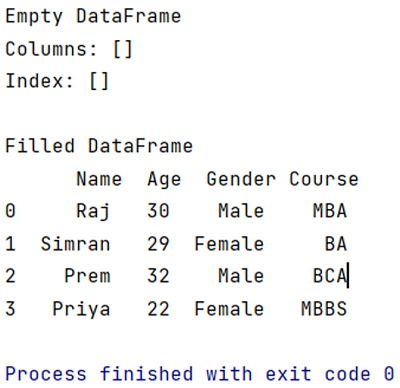# Create an Empty Pandas DataFrame and Fill It

In this tutorial, we will learn how to create an empty Pandas DataFrame and fill it with the data? By Pranit Sharma Last updated : April 12, 2023

## DataFrames

DataFrames are 2-dimensional data structures in Pandas. DataFrames consist of rows, columns, and the data. DataFrame can be created with the help of python dictionaries but in the real world, CSV files are imported and then converted into DataFrames.

## Create an Empty DataFrame

To create an empty Pandas DataFrame, use pandas.DataFrame() method. It creates an DataFrame with no columns or no rows.

Use the following 2 steps syntax to create an empty DataFrame,

### Syntax

```# Import the pandas library
import pandas as pd

# Create empty DataFrame
df = pd.DataFrame()
```

## Fill DataFrame with Data

To fill am empty DataFrame (or, to append the values in a DataFrame), use the column name and assign the set of values directly. Use the following syntax to fill DataFrame,

### Syntax

```df['column1'] = ['val_1','val_2','val_3','val_4']
```

Let us understand with the help of an example.

## Example to Create an Empty Pandas DataFrame and Fill It

```# Importing pandas package
import pandas as pd

# Creating an empty DataFrame
df = pd.DataFrame()

# Printing an empty DataFrame
print(df)

# Appending new columns and rows
df['Name'] = ['Raj','Simran','Prem','Priya']
df['Age'] = [30,29,32,22]
df['Gender'] = ['Male','Female','Male','Female']
df['Course'] = ['MBA','BA','BCA','MBBS']

# After creating rows and columns,
# we will now print this DataFrame
print("\nFilled DataFrame\n",df)
```

### Output

```Empty DataFrame
Columns: []
Index: []

Filled DataFrame
Name  Age  Gender Course
0     Raj   30    Male    MBA
1  Simran   29  Female     BA
2    Prem   32    Male    BCA
3   Priya   22  Female   MBBS
```

### Output (Screenshot)